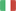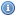EnglishItalianoVisitatore
Torna allaHomepage...

###Generali:

• Dipartimento: Scienze Matematiche, Fisiche E Naturali
• Tipologia: Corso Di Laurea Dm.270/04
• Corso di Laurea: Chimica
• Settore Ministeriale: MAT/05
• Codice di verbalizzazione: 8067370
• Metodi di insegnamento: Frontale
• Metodi di valutazione: Scritto E Orale
• Prerequisiti: Basic Mathematics as taught in every high school
• Obiettivi: Students will learn basic notions of differential and integral calculus (for real-valued functions of a real variable), and of linear algebra. They will be able to apply them to solve simple ordinary differential equations and to study systems of linear equations.KNOWLEDGE AND UNDERSTANDING: Understanding of basic concepts of infinitesimal calculus and linear algebra, with some examples of mathematical formalization. APPLYING KNOWLEDGE AND UNDERSTANDING: Students will be able to compute simple limits, derivatives, integrals, differential equations, and linear systems; students will be able to recognize the advantages of mathematical formalization and of the application of mathematical methods. MAKING JUDGEMENTS: Students will be able to figure out the solution method for simple mathematical problems. COMMUNICATION SKILLS: Students will learn how to explain the mathematical procedure chosen to solve a problem. LEARNING SKILLS: Students will learn how to distinguish the essential features of a mathematical problem and how to study from a textbook effectively.
• Ricevimento: Mar 13-15

###Didattica:

• A.A.: 2020/2021
• Canale: UNICO
• Crediti (CFU): 9
• Obbligo di Frequenza: No

###Classi virtuali:

• Nome classe: DAMASCELLI-8067370-ANALISI_MATEMATICA_I